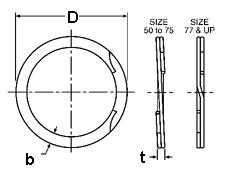### RRN-077

Display Units: inches |  metric
Request Quick Quote for this part.
 Ring Specs (D) Free Diameter: 0.836 +0.013 / -0.000 in. (t) Thickness: 0.042 +0.002 / -0.002 in. (b) Radial Wall: 0.075 +0.003 / -0.005 in. Groove Specs: (B) Application Diameter: 0.777 in. (G) Groove Diameter: 0.825 +0.003 / -0.003 in. (W) Groove Width: 0.046 +0.003 / -0.000 in. Groove Depth: 0.024 in. Other Specs Approximate Weight per 1000: (m) Material Thickness: 0.020 in. (N) Ring Number of Turns: 2.000 (Pg) Theoretical Thrust Load Capacity - Groove Yield:Notes: Yield Strength of Groove Material (Ys): 45,000 psi. Calculated using a safety factor (K) of 2Equation:Pg = [ B * d * Ys * pi ] / K    = [ (0.777 in.) * (0.024 in.) * (45,000 psi) * 3.14 ] / 2 1,317 lbs. (Pr) Theoretical Thrust Load Capacity - Ring Shear:Notes: Ring Material: Carbon Spring Steel (SAE 1070-1090). Shear Strength of Ring Material* (Ss): 138,000 psi. Calculated using a safety factor (K) of 3Equation:Pr = [ B * t * Ss * pi ] / K    = [ (0.777 in.) * (0.042 in.) * (138,000 psi) * 3.14 ] / 3*Shear Strength of Material value is for Carbon Spring Steel material only. 4,713 lbs. Industry Equivalent Part Number(s):RRN-077, KG-77, WHM-77, RRN-77